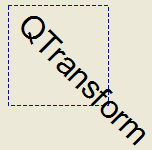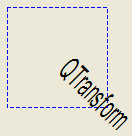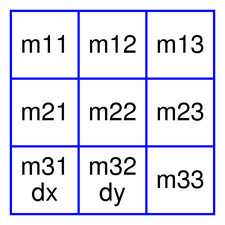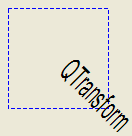# QTransform#

The `QTransform` class specifies 2D transformations of a coordinate system. More

## Synopsis#

### Static functions#

Note

This documentation may contain snippets that were automatically translated from C++ to Python. We always welcome contributions to the snippet translation. If you see an issue with the translation, you can also let us know by creating a ticket on https:/bugreports.qt.io/projects/PYSIDE

## Detailed Description#

Warning

This section contains snippets that were automatically translated from C++ to Python and may contain errors.

A transformation specifies how to translate, scale, shear, rotate or project the coordinate system, and is typically used when rendering graphics.

A `QTransform` object can be built using the `setMatrix()` , `scale()` , `rotate()` , `translate()` and `shear()` functions. Alternatively, it can be built by applying `basic matrix operations` . The matrix can also be defined when constructed, and it can be reset to the identity matrix (the default) using the `reset()` function.

The `QTransform` class supports mapping of graphic primitives: A given point, line, polygon, region, or painter path can be mapped to the coordinate system defined by this matrix using the `map()` function. In case of a rectangle, its coordinates can be transformed using the `mapRect()` function. A rectangle can also be transformed into a polygon (mapped to the coordinate system defined by this matrix), using the `mapToPolygon()` function.

`QTransform` provides the `isIdentity()` function which returns `true` if the matrix is the identity matrix, and the `isInvertible()` function which returns `true` if the matrix is non-singular (i.e. AB = BA = I). The `inverted()` function returns an inverted copy of this matrix if it is invertible (otherwise it returns the identity matrix), and `adjoint()` returns the matrix’s classical adjoint. In addition, `QTransform` provides the `determinant()` function which returns the matrix’s determinant.

Finally, the `QTransform` class supports matrix multiplication, addition and subtraction, and objects of the class can be streamed as well as compared.

### Rendering Graphics#

When rendering graphics, the matrix defines the transformations but the actual transformation is performed by the drawing routines in `QPainter` .

By default, `QPainter` operates on the associated device’s own coordinate system. The standard coordinate system of a `QPaintDevice` has its origin located at the top-left position. The x values increase to the right; y values increase downward. For a complete description, see the coordinate system documentation.

`QPainter` has functions to translate, scale, shear and rotate the coordinate system without using a `QTransform` . For example:```def paintEvent(self, arg__0): painter = QPainter(self) painter.setPen(QPen(Qt.blue, 1, Qt.DashLine)) painter.drawRect(0, 0, 100, 100) painter.rotate(45) painter.setFont(QFont("Helvetica", 24)) painter.setPen(QPen(Qt.black, 1)) painter.drawText(20, 10, "QTransform") ```

Although these functions are very convenient, it can be more efficient to build a `QTransform` and call `setTransform()` if you want to perform more than a single transform operation. For example:```def paintEvent(self, arg__0): painter = QPainter(self) painter.setPen(QPen(Qt.blue, 1, Qt.DashLine)) painter.drawRect(0, 0, 100, 100) transform = QTransform() transform.translate(50, 50) transform.rotate(45) transform.scale(0.5, 1.0) painter.setTransform(transform) painter.setFont(QFont("Helvetica", 24)) painter.setPen(QPen(Qt.black, 1)) painter.drawText(20, 10, "QTransform") ```

### Basic Matrix Operations#A `QTransform` object contains a 3 x 3 matrix. The `m31` (`dx`) and `m32` (`dy`) elements specify horizontal and vertical translation. The `m11` and `m22` elements specify horizontal and vertical scaling. The `m21` and `m12` elements specify horizontal and vertical shearing. And finally, the `m13` and `m23` elements specify horizontal and vertical projection, with `m33` as an additional projection factor.

`QTransform` transforms a point in the plane to another point using the following formulas:

```x' = m11x + m21y + dx
y' = m22y + m12x + dy
if not isAffine():
w' = m13x + m23y + m33
x' /= w'
y' /= w'
```

The point (x, y) is the original point, and (x’, y’) is the transformed point. (x’, y’) can be transformed back to (x, y) by performing the same operation on the `inverted()` matrix.

The various matrix elements can be set when constructing the matrix, or by using the `setMatrix()` function later on. They can also be manipulated using the `translate()` , `rotate()` , `scale()` and `shear()` convenience functions. The currently set values can be retrieved using the `m11()` , `m12()` , `m13()` , `m21()` , `m22()` , `m23()` , `m31()` , `m32()` , `m33()` , `dx()` and `dy()` functions.

Translation is the simplest transformation. Setting `dx` and `dy` will move the coordinate system `dx` units along the X axis and `dy` units along the Y axis. Scaling can be done by setting `m11` and `m22`. For example, setting `m11` to 2 and `m22` to 1.5 will double the height and increase the width by 50%. The identity matrix has `m11`, `m22`, and `m33` set to 1 (all others are set to 0) mapping a point to itself. Shearing is controlled by `m12` and `m21`. Setting these elements to values different from zero will twist the coordinate system. Rotation is achieved by setting both the shearing factors and the scaling factors. Perspective transformation is achieved by setting both the projection factors and the scaling factors.

### Combining Transforms#

Here’s the combined transformations example using basic matrix operations:```def paintEvent(self, arg__0): a = qDegreesToRadians(45.0) sina = sin(a) cosa = cos(a) scale = QTransform(0.5, 0, 0, 1.0, 0, 0) rotate = QTransform(cosa, sina, -sina, cosa, 0, 0) translate = QTransform(1, 0, 0, 1, 50.0, 50.0) transform = scale * rotate * translate painter = QPainter(self) painter.setPen(QPen(Qt.blue, 1, Qt.DashLine)) painter.drawRect(0, 0, 100, 100) painter.setTransform(transform) painter.setFont(QFont("Helvetica", 24)) painter.setPen(QPen(Qt.black, 1)) painter.drawText(20, 10, "QTransform") ```

The combined transform first scales each operand, then rotates it, and finally translates it, just as in the order in which the product of its factors is written. This means the point to which the transforms are applied is implicitly multiplied on the left with the transform to its right.

### Relation to Matrix Notation#

The matrix notation in `QTransform` is the transpose of a commonly-taught convention which represents transforms and points as matrices and vectors. That convention multiplies its matrix on the left and column vector to the right. In other words, when several transforms are applied to a point, the right-most matrix acts directly on the vector first. Then the next matrix to the left acts on the result of the first operation - and so on. As a result, that convention multiplies the matrices that make up a composite transform in the reverse of the order in `QTransform` , as you can see in `Combining Transforms` . Transposing the matrices, and combining them to the right of a row vector that represents the point, lets the matrices of transforms appear, in their product, in the order in which we think of the transforms being applied to the point.

class PySide6.QtGui.QTransform#

PySide6.QtGui.QTransform(other)

PySide6.QtGui.QTransform(h11, h12, h13, h21, h22, h23, h31, h32, h33)

PySide6.QtGui.QTransform(h11, h12, h21, h22, dx, dy)

Parameters:
• dy – float

• h13 – float

• h31 – float

• dx – float

• h33 – float

• h23 – float

• h21 – float

• h11 – float

• h22 – float

• h32 – float

• h12 – float

Constructs an identity matrix.

All elements are set to zero except `m11` and `m22` (specifying the scale) and `m33` which are set to 1.

Constructs a matrix with the elements, `m11`, `m12`, `m13`, `m21`, `m22`, `m23`, `m31`, `m32`, `m33`.

Constructs a matrix with the elements, `m11`, `m12`, `m21`, `m22`, `dx` and `dy`.

PySide6.QtGui.QTransform.TransformationType#

Constant

Description

QTransform.TxNone

QTransform.TxTranslate

QTransform.TxScale

QTransform.TxRotate

QTransform.TxShear

QTransform.TxProject

PySide6.QtGui.QTransform.__reduce__()#
Return type:

object

PySide6.QtGui.QTransform.__repr__()#
Return type:

object

Return type:

`PySide6.QtGui.QTransform`

Returns the adjoint of this matrix.

PySide6.QtGui.QTransform.determinant()#
Return type:

float

Returns the matrix’s determinant.

PySide6.QtGui.QTransform.dx()#
Return type:

float

Returns the horizontal translation factor.

`m31()` `translate()` `Basic Matrix Operations`

PySide6.QtGui.QTransform.dy()#
Return type:

float

Returns the vertical translation factor.

`translate()` `Basic Matrix Operations`

static PySide6.QtGui.QTransform.fromScale(dx, dy)#
Parameters:
• dx – float

• dy – float

Return type:

`PySide6.QtGui.QTransform`

Creates a matrix which corresponds to a scaling of `sx` horizontally and `sy` vertically. This is the same as `QTransform()` .scale(sx, sy) but slightly faster.

static PySide6.QtGui.QTransform.fromTranslate(dx, dy)#
Parameters:
• dx – float

• dy – float

Return type:

`PySide6.QtGui.QTransform`

Creates a matrix which corresponds to a translation of `dx` along the x axis and `dy` along the y axis. This is the same as `QTransform()` .translate(dx, dy) but slightly faster.

PySide6.QtGui.QTransform.inverted()#
Return type:

PyTuple

Returns an inverted copy of this matrix.

If the matrix is singular (not invertible), the returned matrix is the identity matrix. If `invertible` is valid (i.e. not 0), its value is set to true if the matrix is invertible, otherwise it is set to false.

PySide6.QtGui.QTransform.isAffine()#
Return type:

bool

Returns `true` if the matrix represent an affine transformation, otherwise returns `false`.

PySide6.QtGui.QTransform.isIdentity()#
Return type:

bool

Returns `true` if the matrix is the identity matrix, otherwise returns `false`.

PySide6.QtGui.QTransform.isInvertible()#
Return type:

bool

Returns `true` if the matrix is invertible, otherwise returns `false`.

PySide6.QtGui.QTransform.isRotating()#
Return type:

bool

Returns `true` if the matrix represents some kind of a rotating transformation, otherwise returns `false`.

Note

A rotation transformation of 180 degrees and/or 360 degrees is treated as a scaling transformation.

PySide6.QtGui.QTransform.isScaling()#
Return type:

bool

Returns `true` if the matrix represents a scaling transformation, otherwise returns `false`.

PySide6.QtGui.QTransform.isTranslating()#
Return type:

bool

Returns `true` if the matrix represents a translating transformation, otherwise returns `false`.

PySide6.QtGui.QTransform.m11()#
Return type:

float

Returns the horizontal scaling factor.

`scale()` `Basic Matrix Operations`

PySide6.QtGui.QTransform.m12()#
Return type:

float

Returns the vertical shearing factor.

`shear()` `Basic Matrix Operations`

PySide6.QtGui.QTransform.m13()#
Return type:

float

Returns the horizontal projection factor.

`translate()` `Basic Matrix Operations`

PySide6.QtGui.QTransform.m21()#
Return type:

float

Returns the horizontal shearing factor.

`shear()` `Basic Matrix Operations`

PySide6.QtGui.QTransform.m22()#
Return type:

float

Returns the vertical scaling factor.

`scale()` `Basic Matrix Operations`

PySide6.QtGui.QTransform.m23()#
Return type:

float

Returns the vertical projection factor.

`translate()` `Basic Matrix Operations`

PySide6.QtGui.QTransform.m31()#
Return type:

float

Returns the horizontal translation factor.

`dx()` `translate()` `Basic Matrix Operations`

PySide6.QtGui.QTransform.m32()#
Return type:

float

Returns the vertical translation factor.

`dy()` `translate()` `Basic Matrix Operations`

PySide6.QtGui.QTransform.m33()#
Return type:

float

Returns the division factor.

`translate()` `Basic Matrix Operations`

PySide6.QtGui.QTransform.map(x, y)#
Parameters:
• x – float

• y – float

Warning

This section contains snippets that were automatically translated from C++ to Python and may contain errors.

Maps the given coordinates `x` and `y` into the coordinate system defined by this matrix. The resulting values are put in *``tx`` and *``ty``, respectively.

The coordinates are transformed using the following formulas:

```x' = m11x + m21y + dx
y' = m22y + m12x + dy
if not isAffine():
w' = m13x + m23y + m33
x' /= w'
y' /= w'
```

The point (x, y) is the original point, and (x’, y’) is the transformed point.

`Basic Matrix Operations`

PySide6.QtGui.QTransform.map(r)
Parameters:
Return type:

`PySide6.QtGui.QRegion`

Creates and returns a `QRegion` object that is a copy of the given `region`, mapped into the coordinate system defined by this matrix.

Calling this method can be rather expensive if rotations or shearing are used.

PySide6.QtGui.QTransform.map(a)
Parameters:
Return type:

`PySide6.QtGui.QPolygonF`

Creates and returns a `QPolygonF` object that is a copy of the given `polygon`, mapped into the coordinate system defined by this matrix.

PySide6.QtGui.QTransform.map(a)
Parameters:
Return type:

`PySide6.QtGui.QPolygon`

Creates and returns a `QPolygon` object that is a copy of the given `polygon`, mapped into the coordinate system defined by this matrix. Note that the transformed coordinates are rounded to the nearest integer.

PySide6.QtGui.QTransform.map(p)
Parameters:
Return type:

`PySide6.QtCore.QPoint`

Creates and returns a `QPoint` object that is a copy of the given `point`, mapped into the coordinate system defined by this matrix. Note that the transformed coordinates are rounded to the nearest integer.

PySide6.QtGui.QTransform.map(p)
Parameters:
Return type:

`PySide6.QtCore.QPointF`

Creates and returns a `QPointF` object that is a copy of the given point, `p`, mapped into the coordinate system defined by this matrix.

PySide6.QtGui.QTransform.map(l)
Parameters:
Return type:

`PySide6.QtCore.QLine`

Creates and returns a `QLineF` object that is a copy of the given line, `l`, mapped into the coordinate system defined by this matrix.

PySide6.QtGui.QTransform.map(l)
Parameters:
Return type:

`PySide6.QtCore.QLineF`

Creates and returns a `QLine` object that is a copy of the given `line`, mapped into the coordinate system defined by this matrix. Note that the transformed coordinates are rounded to the nearest integer.

PySide6.QtGui.QTransform.map(p)
Parameters:
Return type:

`PySide6.QtGui.QPainterPath`

Creates and returns a `QPainterPath` object that is a copy of the given `path`, mapped into the coordinate system defined by this matrix.

PySide6.QtGui.QTransform.mapRect(arg__1)#
Parameters:
Return type:

`PySide6.QtCore.QRect`

Creates and returns a `QRect` object that is a copy of the given `rectangle`, mapped into the coordinate system defined by this matrix. Note that the transformed coordinates are rounded to the nearest integer.

PySide6.QtGui.QTransform.mapRect(arg__1)
Parameters:
Return type:

`PySide6.QtCore.QRectF`

Warning

This section contains snippets that were automatically translated from C++ to Python and may contain errors.

Creates and returns a `QRectF` object that is a copy of the given `rectangle`, mapped into the coordinate system defined by this matrix.

The rectangle’s coordinates are transformed using the following formulas:

```x' = m11x + m21y + dx
y' = m22y + m12x + dy
if not isAffine():
w' = m13x + m23y + m33
x' /= w'
y' /= w'
```

If rotation or shearing has been specified, this function returns the bounding rectangle. To retrieve the exact region the given `rectangle` maps to, use the `mapToPolygon()` function instead.

`mapToPolygon()` `Basic Matrix Operations`

PySide6.QtGui.QTransform.mapToPolygon(r)#
Parameters:
Return type:

`PySide6.QtGui.QPolygon`

Warning

This section contains snippets that were automatically translated from C++ to Python and may contain errors.

Creates and returns a `QPolygon` representation of the given `rectangle`, mapped into the coordinate system defined by this matrix.

The rectangle’s coordinates are transformed using the following formulas:

```x' = m11x + m21y + dx
y' = m22y + m12x + dy
if not isAffine():
w' = m13x + m23y + m33
x' /= w'
y' /= w'
```

Polygons and rectangles behave slightly differently when transformed (due to integer rounding), so `matrix.map(QPolygon(rectangle))` is not always the same as `matrix.mapToPolygon(rectangle)`.

`mapRect()` `Basic Matrix Operations`

PySide6.QtGui.QTransform.__ne__(arg__1)#
Parameters:
Return type:

bool

Returns `true` if this matrix is not equal to the given `matrix`, otherwise returns `false`.

PySide6.QtGui.QTransform.__mul__(o)#
Parameters:
Return type:

`PySide6.QtGui.QTransform`

Returns the result of multiplying this matrix by the given `matrix`.

Note that matrix multiplication is not commutative, i.e. a*b != b*a.

PySide6.QtGui.QTransform.__mul__(n)
Parameters:

n – float

Return type:

`PySide6.QtGui.QTransform`

PySide6.QtGui.QTransform.__imul__(arg__1)#
Parameters:
Return type:

`PySide6.QtGui.QTransform`

Returns the result of multiplying this matrix by the given `matrix`.

PySide6.QtGui.QTransform.__imul__(div)
Parameters:

div – float

Return type:

`PySide6.QtGui.QTransform`

Returns the result of performing an element-wise multiplication of this matrix with the given `scalar`.

Parameters:

n – float

Return type:

`PySide6.QtGui.QTransform`

Parameters:

div – float

Return type:

`PySide6.QtGui.QTransform`

Returns the matrix obtained by adding the given `scalar` to each element of this matrix.

PySide6.QtGui.QTransform.__sub__(n)#
Parameters:

n – float

Return type:

`PySide6.QtGui.QTransform`

PySide6.QtGui.QTransform.__isub__(div)#
Parameters:

div – float

Return type:

`PySide6.QtGui.QTransform`

Returns the matrix obtained by subtracting the given `scalar` from each element of this matrix.

PySide6.QtGui.QTransform.__div__(n)#
Parameters:

n – float

Return type:

`PySide6.QtGui.QTransform`

PySide6.QtGui.QTransform.__idiv__(div)#
Parameters:

div – float

Return type:

`PySide6.QtGui.QTransform`

Returns the result of performing an element-wise division of this matrix by the given `scalar`.

PySide6.QtGui.QTransform.__eq__(arg__1)#
Parameters:
Return type:

bool

Returns `true` if this matrix is equal to the given `matrix`, otherwise returns `false`.

Parameters:
Return type:

object

Parameters:
Return type:

bool

Creates a transformation matrix, `trans`, that maps a four-sided polygon, `one`, to another four-sided polygon, `two`. Returns `true` if the transformation is possible; otherwise returns false.

This is a convenience method combining `quadToSquare()` and `squareToQuad()` methods. It allows the input quad to be transformed into any other quad.

Parameters:
Return type:

object

Parameters:
Return type:

bool

Creates a transformation matrix, `trans`, that maps a four-sided polygon, `quad`, to a unit square. Returns `true` if the transformation is constructed or false if such a transformation does not exist.

PySide6.QtGui.QTransform.reset()#

Resets the matrix to an identity matrix, i.e. all elements are set to zero, except `m11` and `m22` (specifying the scale) and `m33` which are set to 1.

`QTransform()` `isIdentity()` `Basic Matrix Operations`

PySide6.QtGui.QTransform.rotate(a[, axis=Qt.ZAxis])#
Parameters:
Return type:

`PySide6.QtGui.QTransform`

Rotates the coordinate system counterclockwise by the given angle `a` about the specified `axis` at distance 1024.0 from the screen and returns a reference to the matrix.

Note that if you apply a `QTransform` to a point defined in widget coordinates, the direction of the rotation will be clockwise because the y-axis points downwards.

The angle is specified in degrees.

PySide6.QtGui.QTransform.rotate(a, axis, distanceToPlane)
Parameters:
Return type:

`PySide6.QtGui.QTransform`

Rotates the coordinate system counterclockwise by the given angle `a` about the specified `axis` at distance `distanceToPlane` from the screen and returns a reference to the matrix.

Note that if you apply a `QTransform` to a point defined in widget coordinates, the direction of the rotation will be clockwise because the y-axis points downwards.

The angle is specified in degrees. If `distanceToPlane` is zero, it will be ignored. This is suitable for implementing orthographic projections where the z coordinate should be dropped rather than projected.

Parameters:
Return type:

`PySide6.QtGui.QTransform`

Rotates the coordinate system counterclockwise by the given angle `a` about the specified `axis` at distance `distanceToPlane` from the screen and returns a reference to the matrix.

Note that if you apply a `QTransform` to a point defined in widget coordinates, the direction of the rotation will be clockwise because the y-axis points downwards.

The angle is specified in radians. If `distanceToPlane` is zero, it will be ignored. This is suitable for implementing orthographic projections where the z coordinate should be dropped rather than projected.

Parameters:
Return type:

`PySide6.QtGui.QTransform`

Rotates the coordinate system counterclockwise by the given angle `a` about the specified `axis` at distance 1024.0 from the screen and returns a reference to the matrix.

Note that if you apply a `QTransform` to a point defined in widget coordinates, the direction of the rotation will be clockwise because the y-axis points downwards.

The angle is specified in radians.

PySide6.QtGui.QTransform.scale(sx, sy)#
Parameters:
• sx – float

• sy – float

Return type:

`PySide6.QtGui.QTransform`

Scales the coordinate system by `sx` horizontally and `sy` vertically, and returns a reference to the matrix.

PySide6.QtGui.QTransform.setMatrix(m11, m12, m13, m21, m22, m23, m31, m32, m33)#
Parameters:
• m11 – float

• m12 – float

• m13 – float

• m21 – float

• m22 – float

• m23 – float

• m31 – float

• m32 – float

• m33 – float

Sets the matrix elements to the specified values, `m11`, `m12`, `m13` `m21`, `m22`, `m23` `m31`, `m32` and `m33`. Note that this function replaces the previous values. `QTransform` provides the `translate()` , `rotate()` , `scale()` and `shear()` convenience functions to manipulate the various matrix elements based on the currently defined coordinate system.

`QTransform()`

PySide6.QtGui.QTransform.shear(sh, sv)#
Parameters:
• sh – float

• sv – float

Return type:

`PySide6.QtGui.QTransform`

Shears the coordinate system by `sh` horizontally and `sv` vertically, and returns a reference to the matrix.

Parameters:
Return type:

object

Parameters:
Return type:

bool

Creates a transformation matrix, `trans`, that maps a unit square to a four-sided polygon, `quad`. Returns `true` if the transformation is constructed or false if such a transformation does not exist.

PySide6.QtGui.QTransform.translate(dx, dy)#
Parameters:
• dx – float

• dy – float

Return type:

`PySide6.QtGui.QTransform`

Moves the coordinate system `dx` along the x axis and `dy` along the y axis, and returns a reference to the matrix.

PySide6.QtGui.QTransform.transposed()#
Return type:

`PySide6.QtGui.QTransform`

Returns the transpose of this matrix.

PySide6.QtGui.QTransform.type()#
Return type:

`TransformationType`

Returns the transformation type of this matrix.

The transformation type is the highest enumeration value capturing all of the matrix’s transformations. For example, if the matrix both scales and shears, the type would be `TxShear`, because `TxShear` has a higher enumeration value than `TxScale`.

Knowing the transformation type of a matrix is useful for optimization: you can often handle specific types more optimally than handling the generic case.